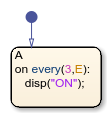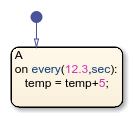# every

Execute chart at regular intervals

## Syntax

``every(n,E)``
``every(n,tick)``
``every(n,sec)``

## Description

example

````every(n,E)` returns `true` at every `n`th occurrence of the event `E` since the associated state became active. Otherwise, the operator returns `false`.```

example

````every(n,tick)` returns `true` at every `n`th time that the chart wakes up since the associated state became active. Otherwise, the operator returns `false`.The implicit event `tick` is not supported when a Stateflow® chart in a Simulink® model has input events.```

example

````every(n,sec)` returns `true` every `n` seconds since the associated state became active. Otherwise, the operator returns `false`.In standalone charts in MATLAB®, specify `n` with a value greater than or equal to `0.001`. The operator creates a MATLAB `timer` object that generates an implicit event to wake up the chart. MATLAB `timer` objects are limited to 1 millisecond precision. For more information, see Events in Standalone Charts. The `timer` object is created when the chart finishes executing the `entry` actions of the associated state and its substates. For subsequent iterations, the `timer` object is reset when the chart finishes executing the `during` actions of the associated state and its substates. If you specify `n` as an expression whose value changes during chart execution, the chart adjusts the temporal delay only when the `timer` object is reset.The `timer` object starts running at the end of the chart step when the associated state becomes active. This step can include the execution of other parallel states in the chart.If the chart is processing another operation when it receives the implicit event from the `timer` object, the chart queues the event. When the current step is completed, the chart processes the event and resets the timer object for the next iteration.If the state associated with the temporal logic operator becomes inactive before the chart processes the implicit event, the event does not wake up the chart. ```

Note

Using `every` as an absolute-time temporal logic operator is supported only in standalone charts in MATLAB. In charts in Simulink models, use an outer self-loop transition with the `after` operator instead. For more information, see Do Not Use every for Absolute-Time Temporal Logic in Charts in Simulink Models.

## Examples

expand all

Display a status message when the chart processes every third broadcast of the event `E` after the state became active.

``````on every(3,E): disp("ON");``````Transition out of the associated state when the chart processes every fifth broadcast of the event `E` after the state became active.

`every(5,E)`Transition out of the associated state every seventh `tick` event since the state became active, but only if the variable `temp` is greater than 98.6.

`every(7,tick)[temp > 98.6]`Increment the `temp` variable by 5 every 12.3 seconds that the state is active.

In standalone charts in MATLAB, enter:

``````on every(12.3,sec): temp = temp+5;``````Using `every` as an absolute-time temporal logic operator is not supported in charts in Simulink models.

## Tips

• You can use quotation marks to enclose the keywords `'tick'` and `'sec'`. For example, `every(5,'tick')` is equivalent to `every(5,tick)`.

• The Stateflow chart resets the counter used by the `every` operator each time the associated state reactivates.

• Standalone charts in MATLAB define absolute-time temporal logic in terms of wall-clock time, which is limited to 1 millisecond precision.

## Version History

Introduced in R2014b